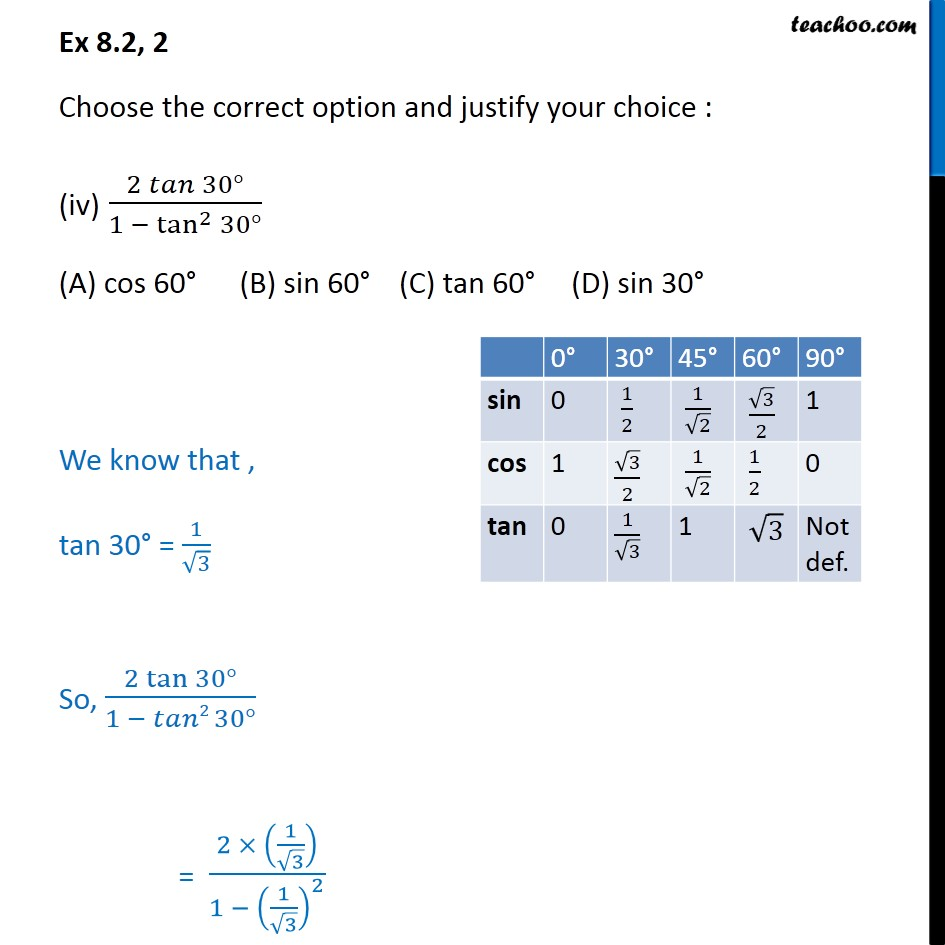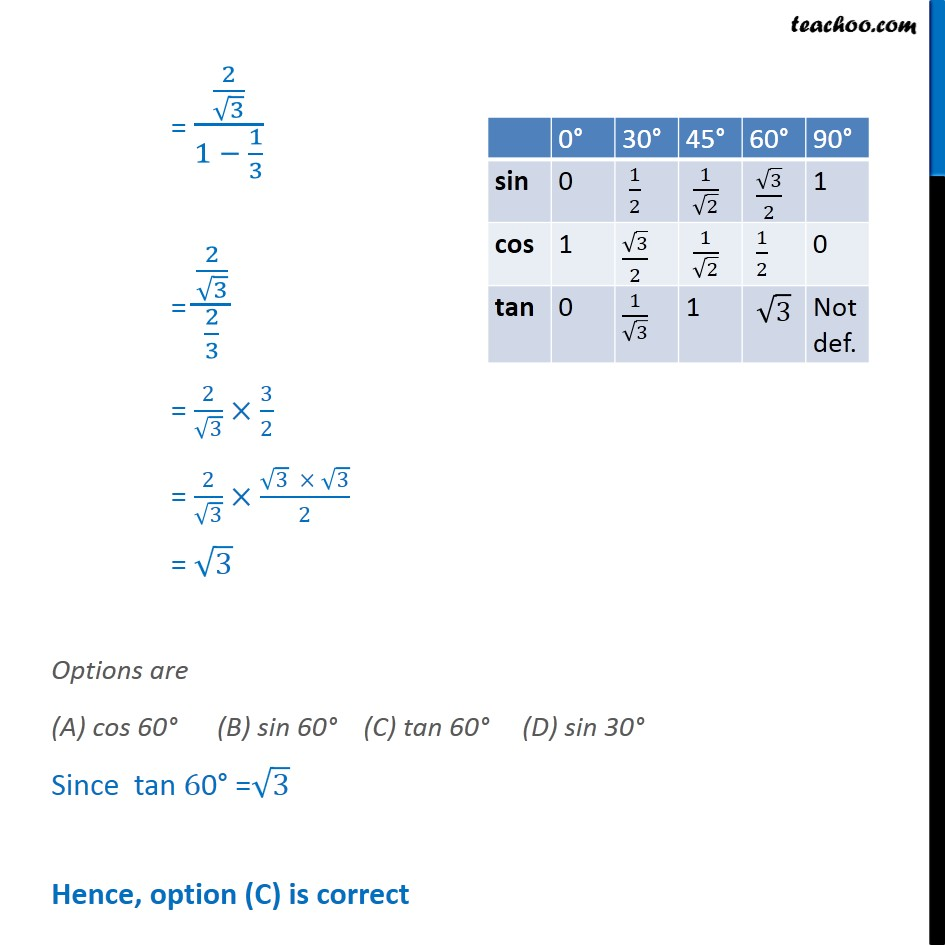1. Chapter 8 Class 10 Introduction to Trignometry (Term 1)
2. Serial order wise
3. Ex 8.2

Transcript

Ex 8.2, 2 Choose the correct option and justify your choice : (iv) (2 𝑡𝑎𝑛⁡〖30°〗)/(1 − tan^2⁡〖30°〗 ) (A) cos 60° (B) sin 60° (C) tan 60° (D) sin 30° We know that , tan "30°" = 1/√3 So, (2 tan⁡〖30°〗)/(1 − 𝑡𝑎𝑛2 30°) = (2 × (1/√3))/(1 − (1/√3)^2 ) = (2/√3)/(1 − 1/3) = (2/√3)/(2/3) = 2/√3×3/2 = 2/√3×(√3 × √3)/2 = √3 Options are (A) cos 60° (B) sin 60° (C) tan 60° (D) sin 30° Since tan "60°" =√3 Hence, option (C) is correct